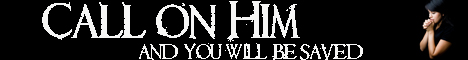Note:  Do not rely on this information. It is very old.

# Statics

Statics is that branch of Dynamics (or Mechanics) which deals with the balancing of forces acting on any body. Although such a balancing of forces causes the body to appear at rest, we must remember that we are never in a position to regard anything as absolutely at rest, We can only deal with relative rest. A body which appears perfectly still with regard to the earth, is at the same time whirling through space with the velocity of our planet, so its condition of rest is only relative. The problem of statics is the discovery of the conditions which determine that any number of forces acting on a body shall produce equilibrium. If two forces O A, 0 B, act at a point O, their resultant is 0 R. [PARALLELOGRAM OF FORCES.] Hence a force R O is exactly sufficient to balance the two forces O A and 0 B. But A R is equal to 0 B, so that the system of forces 0 A, A R, R 0, forming the closed figure 0 A R, is in equilibrium. This proposition can be extended, and it is quite simple to prove that if any number of forces acting at a point can be represented in magnitude and direction by the sides of a closed polygon taken in order, those forces will be in equilibrium. This is known as the Polygon of Forces. If two forces act on a body, this condition can only be fulfilled when the forces act at a point, are equal in magnitude and opposite in direction. If the forces act in a plane at different points of a body, the simplest metbod of arriving at the conditions of equilibrium is to revolve the forces in two directions at right angles to each other. [RESOLUTION OF FORCES.] Then the sum (algebraical) of the forces in each of these two directions must be zero; but this is not all. Since the forces are not acting at a point, they may produce a rotation, and for this not to happen the sum of the moments of the forces [MOMENT] about any point in their plane must also be zero. If forces act on a rigid body in any d1rections whatever, the conditions for equilibrium are that the algebraical sums of the components of the forces about eaoh of three axes at right angles must be zero, and the sums of the moments of the forces about the same three axes must also be zero. An important set of problems in statics is concerned with finding the centres of graviity or mass centres of different bodies, the forces under consideration being in that case the weights of the different particles composing the body. Equilibrium may be stable, unstable, or neutral [STABILITY], and the state of greatest stability is reached when the body possesses the minimum potential energy. [ENERGY.]